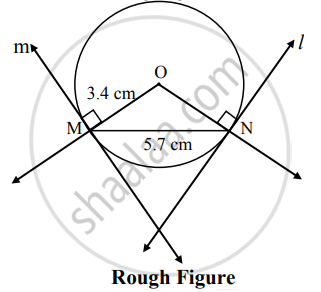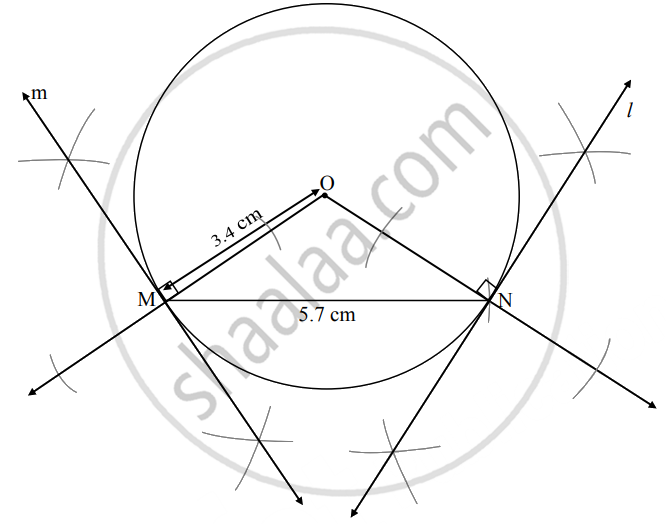# Draw a circle with center O and radius 3.4. Draw a chord MN of length 5.7 cm in a circle. Draw tangents to the circle from point M and N - Geometry

Diagram

Draw a circle with center O and radius 3.4. Draw a chord MN of length 5.7 cm in a circle. Draw tangents to the circle from point M and N

#### SolutionAnalysis:
{:("seg ON" ⊥ "line  l"),("seg OM" ⊥ "line m"):}}  ......[Tangent theorem]

The perpendicular to seg ON and seg OM at points N and M respectively will give the required tangents at N and M.Steps of construction:

1. With center O, draw a circle of radius 3.4 cm
2. Draw chord MN of length 5.7 cm in the circle.
3. Draw rays OM and ON.
4. Draw line l ⊥ ray ON at point N.
5. Draw line m ⊥ ray OM at point M.
6. Lines l and m are the required tangents at points M and N to the circle.
Concept: Construction of a Tangent to the Circle at a Point on the Circle
Is there an error in this question or solution?

Share